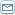# Boolean Algebra

 By: devendra saxenaOne of the primary requirements when dealing with digital circuits is to find ways to make them as simple as possible. This constantly requires that complex logical expressions be reduced to simpler expressions that nevertheless produce the same results under all possible conditions. The simpler expression can then be implemented with a smaller, simpler circuit, which in turn saves the price of the unnecessary gates, reduces the number of gates needed, and reduces the power and the amount of space required by those gates.One tool to reduce logical expressions is the mathematics of logical expressions, introduced by George Boole in 1854 and known today as Boolean Algebra. The rules of Boolean Algebra are simple and straight-forward, and can be applied to any logical expression. The resulting reduced expression can then be readily tested with a Truth Table, to verify that the reduction was valid.This has a very great role to play in abstract algebra. In fact, in abstract algebra, Boolean algebra is an algebraic that captures essential properties of both set operation and logic operations. Specifically, it deals with the set operations of intersection, union, complement and the logic operations of AND, or, NOT. Like any lattice, a Boolean algebra (A, land, lor) gives rise to a partially ordered set (A, â‰?) by defining a â‰? b precisely when a = a land b (which is also equivalent to b = a lor b).In fact one can also define a Boolean algebra to be a distributive lattice with least element 0 and greatest element 1, within which every element x has a complement x such that x land x = 0 and x lor x = 1Here land and lor are used to denote the infimum (meet) and supremum (join) of two elements. Again, if complements in the above sense exist, then they are uniquely determined.The algebraic and the order theoretic perspective can usually be used interchangeably and both are of great use to import results and concepts from both universal algebra and order theory. In many practical examples an ordering relation, conjunction, disjunction, and negation are all naturally available, so that it is straightforward to exploit this relationship. One can also apply general insights from duality in order theory to Boolean algebras. Especially, the order dual of every Boolean algebra, or, equivalently, the algebra obtained by exchanging land and lor, is also a Boolean algebra. In general, any law valid for Boolean algebras can be transformed into another valid, dual law by exchanging 0 with 1, land with lor, and â‰? with â‰?.The operators of Boolean algebra may be represented in various ways. Often they are simply written as AND, OR and NOT. In describing circuits, NAND (Not AND), NOR (Not OR) and XOR may also be used. Mathematicians, engineers, and programmers often use + for OR and ? for AND (since in some ways those operations are analogous to addition and multiplication in other algebraic structures and this notation makes it very easy to get sum of products form for people who are familiar with normal algebra) and represent NOT by a line drawn above the expression being negated. Sometimes, the symbol ~ or ! is used for NOT.Every Boolean algebra (A, land, lor) gives rise to a ring (A, +, *) by defining a + b = (a land b) lor (b land a) (this operation is called symmetric difference in the case of sets and XOR in the case of logic) and a * b = a land b. The zero element of this ring coincides with the 0 of the Boolean algebra; the multiplicative identity element of the ring is the 1 of the Boolean algebra. This ring has the property that a * a = a, for all a in A; rings with this property are called Boolean rings.Conversely, if a Boolean ring A is given, we can turn it into a Boolean algebra by defining x lor y = x + y + xy and x land y = xy. Since these two operations are inverses of each other, we can say that every Boolean ring arises from a Boolean algebra, and vice versa. Furthermore, a map f: A â†’ B is a homomorphism of Boolean algebras if and only if it is a homomorphism of Boolean rings. The categories of Boolean rings and Boolean algebras are equivalent.An ideal of the Boolean algebra A is a subset I such that for all x, y in I we have x lor y in I and for all a in A we have a land x in I. This notion of ideal coincides with the notion of ring ideal in the Boolean ring A. An ideal I of A is called prime if I â‰? A and if a land b in I always implies a in I or b in I. An ideal I of A is called maximal if I â‰? A and if the only ideal properly containing I is A itself. These notions coincide with ring theoretic ones of prime ideal and maximal ideal in the Boolean ring A.The dual of an ideal is a filter. A filter of the Boolean algebra A is a subset p such that for all x, y in p we have x land y in p and for all a in A if a lor x = a then a in p.REPRINT RIGHTS statement: This article is free for republishing by visitors provided the Author Bio box is retained as usual so that all links are Active/Linkable with no syntax changes.